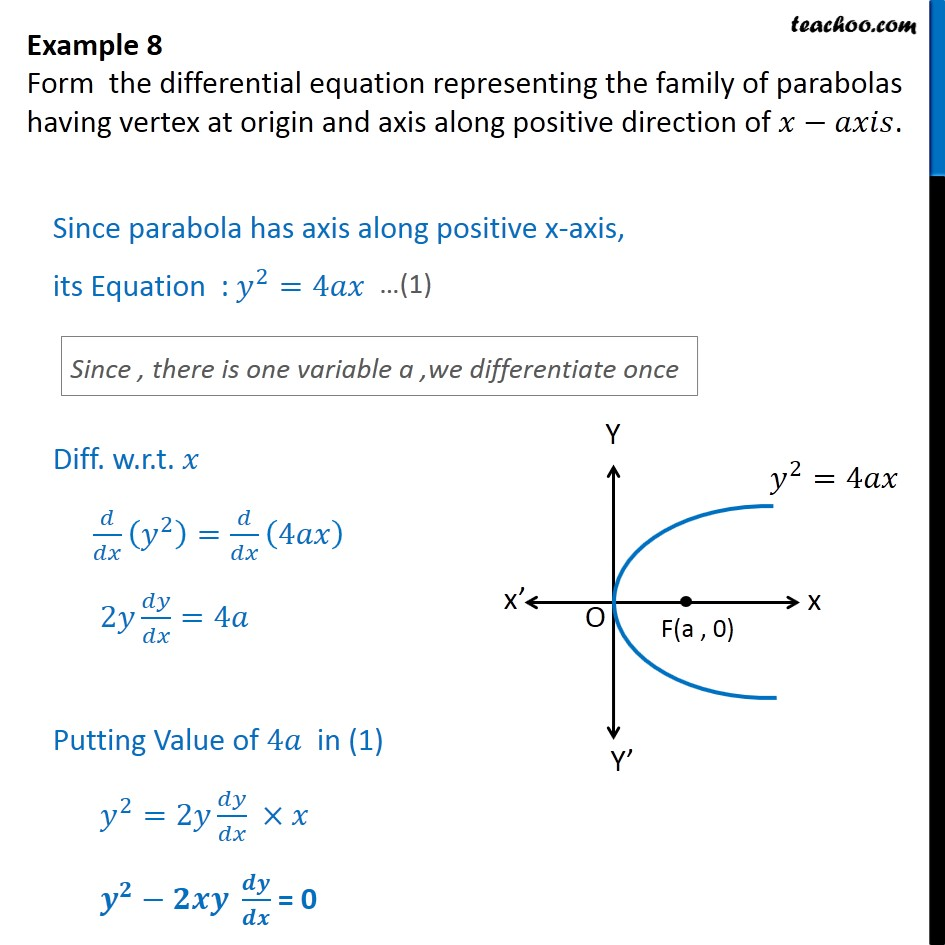Examples

Chapter 9 Class 12 Differential Equations
Serial order wiseLearn in your speed, with individual attention - Teachoo Maths 1-on-1 Class

### Transcript

Question 5 Form the differential equation representing the family of parabolas having vertex at origin and axis along positive direction of . Since parabola has axis along positive x-axis, its Equation : 2 =4 Diff. w.r.t. 2 = 4 2 =4 Putting Value of 4 in (1) 2 =2 = 0